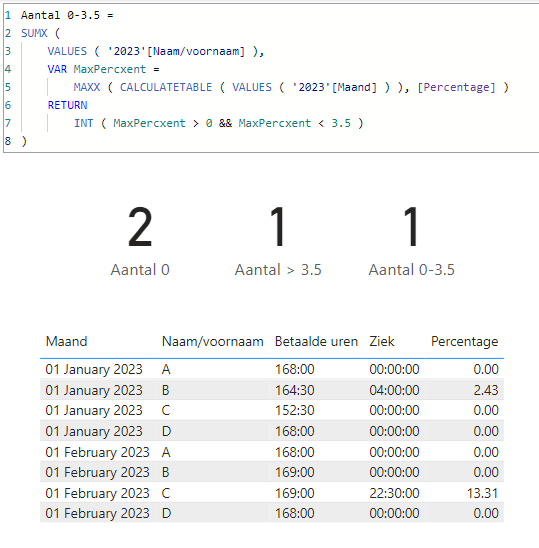cancel
Showing results for
Did you mean:

Fabric is Generally Available. Browse Fabric Presentations. Work towards your Fabric certification with the Cloud Skills Challenge.Frequent Visitor

## Countrows/Distinctcount DAX question

Hi all,

Maybe you could help me with this one.

I'm trying to how many employees are in a certain category of average sickness (for example 0), based on the months selected in the slicer.

I have a calculation in a calculated column of the table where I calculate the percentage of each employee for each month:

Percentage =divide('2023'[Ziek],'2023'[Betaalde uren])

If I do this for only 1 month with:

Aantal 0 = COUNTROWS(FILTER('2023','2023'[Percentage]=0)) it works but when I select more then 1 month it's add up.
But I only want in case of selecting multiple months on the slicer that still have an average percentage of 0.
I tried it with distinctcount but for some reason it gives me the same result.
See below for some data.
Result when selecting january on the slicer should be: 3, result of selecting january and february on the slicer should be 2.

 Maand Naam/voornaam Betaalde uren Ziek Percentage 1-1-2023 A 168:00 0:00 0,00 1-1-2023 B 164:30 4:00 2,43 1-1-2023 C 152:30 0:00 0,00 1-1-2023 D 168:00 0:00 0,00 1-2-2023 A 168:00 0:00 0,00 1-2-2023 B 169:00 0:00 0,00 1-2-2023 C 169:00 22:30 13,31 1-2-2023 D 168:00 0:00 0,00
1 ACCEPTED SOLUTIONSuper User

@meskens
The > 3.5 seems to be working. Not sure why you're facing issues with it. I prepared a quick sample file for testing the 3 formulas.``````Aantal 0 =
SUMX (
VALUES ( '2023'[Naam/voornaam] ),
INT ( MAXX ( CALCULATETABLE ( VALUES ( '2023'[Maand] ) ), [Percentage] ) = 0 )
)``````
``````Aantal > 3.5 =
SUMX (
VALUES ( '2023'[Naam/voornaam] ),
INT ( MAXX ( CALCULATETABLE ( VALUES ( '2023'[Maand] ) ), [Percentage] ) > 3.5 )
)``````
``````Aantal 0-3.5 =
SUMX (
VALUES ( '2023'[Naam/voornaam] ),
VAR MaxPercxent =
MAXX ( CALCULATETABLE ( VALUES ( '2023'[Maand] ) ), [Percentage] )
RETURN
INT ( MaxPercxent > 0 && MaxPercxent < 3.5 )
)``````
6 REPLIES 6Frequent Visitor

Hi @tamerj1 ,

This works great!

One more question, 1 have two more categories:

>0 and <3,5

>= 3,5

However when I change youre code to

SUMX (VALUES ( '2023'[Naam/voornaam] ),INT (MAXX (CALCULATETABLE (VALUES ( '2023'[Maand] )),[Percentage]) >3.5))

It gives no number (I have more date in my own power bi) Should have been 1

When I change the code to

Aantal 0-3.5 = SUMX ( VALUES ( '2023'[Naam/voornaam] ), INT ( MAXX ( CALCULATETABLE ( VALUES ( '2023'[Maand] ) ), [Percentage] ) > 0 && [Percentage]<3.5 ) )
It counts everything > 0 but not <3.5

What am I doing wrong?Super User

@meskens
The > 3.5 seems to be working. Not sure why you're facing issues with it. I prepared a quick sample file for testing the 3 formulas.``````Aantal 0 =
SUMX (
VALUES ( '2023'[Naam/voornaam] ),
INT ( MAXX ( CALCULATETABLE ( VALUES ( '2023'[Maand] ) ), [Percentage] ) = 0 )
)``````
``````Aantal > 3.5 =
SUMX (
VALUES ( '2023'[Naam/voornaam] ),
INT ( MAXX ( CALCULATETABLE ( VALUES ( '2023'[Maand] ) ), [Percentage] ) > 3.5 )
)``````
``````Aantal 0-3.5 =
SUMX (
VALUES ( '2023'[Naam/voornaam] ),
VAR MaxPercxent =
MAXX ( CALCULATETABLE ( VALUES ( '2023'[Maand] ) ), [Percentage] )
RETURN
INT ( MaxPercxent > 0 && MaxPercxent < 3.5 )
)``````Frequent Visitor

Thanks @tamerj1 , all works now. I don't know why in first place it didn't work.

I'll look into your code to understand how it works.Frequent Visitor

Hi  Tamerj1,

I get an error message : Function max only accepts a column reference as an argument.Super User

Ok, I thought it was a column. Please try

Aantal 0 =
SUMX (
VALUES ( '2023'[Naam/voornaam] ),
INT (
MAXX (
CALCULATETABLE (
VALUES ( '2023'[Maand] )
),
[Percentage]
) = 0
)
)Super User

Hi @meskens

Aantal 0 =
SUMX (
VALUES ( '2023'[Naam/voornaam] ),
INT ( CALCULATE ( MAX ( '2023'[Percentage] = 0 ) ) = 0 )
)Announcements#### Power BI Monthly Update - November 2023

Check out the November 2023 Power BI update to learn about new features.#### Fabric Community News unified experience

Read the latest Fabric Community announcements, including updates on Power BI, Synapse, Data Factory and Data Activator.#### The largest Power BI and Fabric virtual conference

130+ sessions, 130+ speakers, Product managers, MVPs, and experts. All about Power BI and Fabric. Attend online or watch the recordings.Top Solution Authors
Top Kudoed Authors
Users online (1,802)